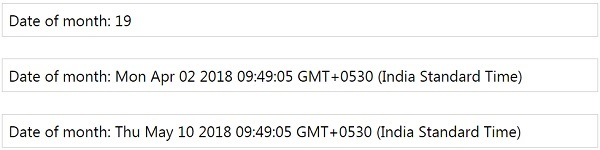# MomentJS - Date of Month

This method will get/set the day of the month. It takes input from 1-31, if greater than the range provided it will add to the next month.

## Syntax

```moment().date(Number);
moment().date();
moment().dates(Number);
moment().dates();
```

## Example

```var m = moment().date(); // gets the current day of the month
var d = moment().date(2); // sets the day of month as shown below
var k = moment().date(40); //sets the day of month which is greater than the
range so the output shows the next month as shown in the output
```

## Outputmomentjs_getter_setter.htm